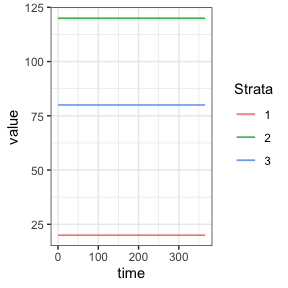# SIS (Susceptible-Infected-Susceptible) Human Model

The SIS (Susceptible-Infected-Susceptible) human model model fulfills the generic interface of the human population component. It is the simplest model of endemic diseases in humans.

# Differential Equations

Let the generalized SIS human model be:

$\dot{X} = \mbox{diag}(bEIR) \cdot (H-X) - rX$

# Equilibrium Solutions

A typical situation when using this model is that $$H$$ (total population size by strata) and $$X$$ (number of infectious persons by strata) are known from census and survey data. Then it is of interest to find the value of $$EIR$$ (Entomological Inoculation Rate) which leads to that prevalence at equilibrium.

$0 = \mbox{diag}(bEIR) \cdot (H-X) - rX$

$rX = \mbox{diag}(b) \cdot \mbox{diag}(EIR) \cdot (H-X)$

$\frac{rX}{H-X} = \mbox{diag}(b) \cdot \mbox{diag}(EIR)$

$\mbox{diag}(1/b) \cdot \left(\frac{rX}{H-X}\right) = EIR$

Note that in the final line, $$EIR$$ is a column vector of dimension $$n$$ due to the operations on the left. Each element gives the per-capita rate at which individuals in that population strata receive potentially infectious bites (summing across all the places they visit).

# Example

library(exDE)
library(deSolve)
library(data.table)
library(ggplot2)

Here we run a simple example with 3 population strata at equilibrium. We use exDE::make_parameters_X_SIS to set up parameters. Please note that this only runs the human population component and that most users should read our fully worked example to run a full simulation.

nStrata <- 3
H <- c(100, 500, 250)
X <- c(20, 120, 80)
b <- 0.55
c <- 0.15
r <- 1/200
Psi <- matrix(data = 1,nrow = 1, ncol = nStrata)

EIR <- diag(1/b, nStrata) %*% ((r*X)/(H-X))

params <- list(
nStrata = nStrata
)
params <- list2env(params)

make_parameters_X_SIS(pars = params, b = b, c = c, r = r, Psi = Psi, X0 = X, H = H)
make_indices(params)

y0 <- rep(0, 3)
y0[params$X_ix] <- X out <- deSolve::ode(y = y0, times = c(0, 365), func = function(t, y, pars, EIR) { list(dXdt(t, y, pars, EIR)) }, parms = params, method = 'lsoda', EIR = as.vector(EIR)) colnames(out)[params$X_ix+1] <- paste0('X_', 1:params\$nStrata)

out <- as.data.table(out)
out <- melt(out, id.vars = 'time')
out[, c("Component", "Strata") := tstrsplit(variable, '_', fixed = TRUE)]
out[, variable := NULL]

ggplot(data = out, mapping = aes(x = time, y = value, color = Strata)) +
geom_line() +
theme_bw()